• ## 层次任务分析

千次阅读 2014-07-21 10:50:24
 　要做好以用户为中心的设计，便要求我们需要更好地了解用户、理解用户。作为交互设计师，在动手画交互稿之前，我们需要理清用户（User）、目标（Goal）、任务（Task）。 　　今天主要探讨任务分析。目前，常用的任务分析方法主要有：层次任务分析（Hierarchical Task Analysis）与认知任务分析（Cognitive Task Analysis）。这里将主要阐述任务分析中的层次任务分析。

什么是层次任务分析？ 　　层次任务分析是一种结构化的客观化地描述任务与其子任务层次体系的方法。在用户体验设计中，层次任务分析用来分析并描述用户如何为达到目标所进行的一系列任务，以及用户与软件系统是如何交互的。

为什么使用层次任务分析？ 　　我们通过层级分析将任务不断拆解，逐级细化用户的任务，直至用户实际的具体操作。随着任务的细化，我们对用户和产品的理解会越来越清晰。然后再通过任务计划（Plan）将子任务进行重组，来勾勒出用户实际的操作流程。 　　当设计全新的产品，层次任务分析可以让你尝试探索用户通过各种不同的方式来完成相同的任务。设计需要能够满足这些用户的行为路径。 对于现有的产品，层次任务分析可以帮助你优化交互设计，使得他们变得更自然、更人性化。

项目实战 　　为了方便大家的理解，这里举一个例子。假设没有竞品，我们就通过用户的任务分析，来设计一个网上书店。
使用简单任务分析 1、用户目标：买到一本所要的书。 2、任务分析 　　根据用户在生活中的行为与互联网特点，对用户的任务分析如下：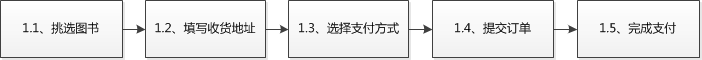3、低保真设计稿为了让用户完成挑选图书的任务，设计师参考了实体书店对于图书的展示方式。设计如下： 　　用户真的能找到所要的图书么？再仔细想想。去书店买书的人，大致可以分为两类： 　　第一类用户，非常明确自己所要购买的图书。生活中，他们通常会直奔某个分类区去寻找自己要的书，或者直接询问店员；第二类用户，不确定自己所要购买的图书。生活中，他们会在书店四处逛逛。有感兴趣的图书，就会购买。 　　根据之前提到的两类用户，对于用户挑选图书可能会有些新的认识。

使用层次任务分析 1、用户目标：买到一本所要的书。 2、任务分析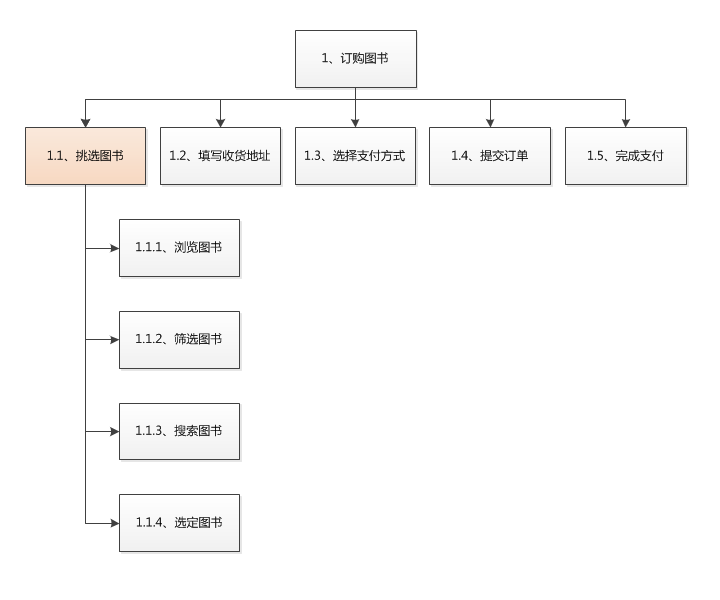我们尝试使用层次任务分析。 　　在任务分析中，我们可以通过任务计划将一个或多个子任务进行组合来描述用户在系统中的实际操作流程。在现实中，用户任务的执行不可能完全严格按照既有编号顺序。为了保证分析的灵活度，通过层级将任务不断拆解，然后再通过任务计划将任务进行重组来勾勒出用户实际的操作流程。 　　用户的主要任务是订购图书。为了完成这个任务，用户的任务计划为：挑选图书 -> 填写收获地址 -> 选择支付方式 -> 提交订单 -> 完成支付。 　　层次任务分析可以让你尝试探索用户通过各种不同的方式来完成相同的任务。我们对子任务进行拆解。此处仅以挑选图书为例。 　　对浏览图书、筛选图书和搜索图书子任务，用户可以任意执行一个或多个，且执行顺序不唯一。挑选图书的用户的任务计划为： a. 浏览图书 -> 选定图书。 b. 浏览图书 -> 搜索图书 -> 选定图书。 c. 筛选图书 -> 搜索图书 -> 筛选图书 -> 选定图书 d. …… 　　由于子任务之间并不是简单的顺序执行流程，所以在设计时，需要考虑让子任务间的流转更容易，满足这一类的任务计划。
3、低保真设计稿设计稿中能够满足不同类型的用户对于浏览、筛选、搜索，这些子任务的任意组合。设计稿此处仅作示意用。 这个设计稿，相信大家能够感受到已经和目前主流网上书店的挑选图书的功能很接近了。缺少的只是一些商业上的思考与运营。

继续细化 　　随着子任务的不断拆分，很快就能找到用户在界面上的实际操作流程。
任务分析：对搜索任务继续拆解。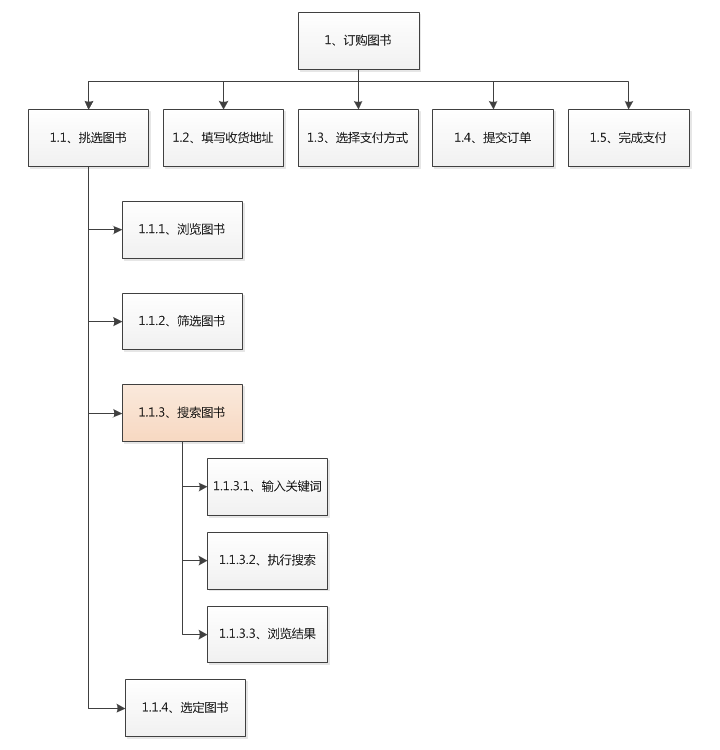根据这一层级的子任务，我们在制作storyboard时会非常轻松。当然，其更好的应用是在完成产品原型后，将实际用户的操作路径与当前任务计划进行比较。通过比较，我们会比较容易找出那些可以优化的点来。

写在最后 　　本文的目的在于介绍在项目中使用层次任务分析，能够让我们对用户、对产品能够思考得更缜密些。层次任务分析主要关注工作中可观察和可编制的任务，很大程度上忽略了整合个人/团队知识、技能和态度等外界因素。另一种，认知任务分析正好弥补这一不足。
展开全文• ## 数据分析简单例子

万次阅读 多人点赞 2018-01-31 16:43:51
数据挖掘是一种技术，它将传统的数据分析方法与处理大量数据的复杂算法相结合。 数据挖掘是在大型数据存储库中，自动地发现有用信息的过程。数据挖掘技术用来探查大型数据库，发现先前未知的有用模式。 数据挖掘...
数据挖掘是一种技术，它将传统的数据分析方法与处理大量数据的复杂算法相结合。
数据挖掘是在大型数据存储库中，自动地发现有用信息的过程。数据挖掘技术用来探查大型数据库，发现先前未知的有用模式。
数据挖掘（Data mining）是一个跨学科的计算机科学分支。它是用人工智能、机器学习、统计学和数据库的交叉方法在相对较大型的数据集中发现模式的计算过程。
数据挖掘的基本任务
利用分类与预测、聚类分析、关联规则、时序模式、偏差检测、智能推荐等方法，帮助企业提取数据中蕴含的商业价值，提高企业的竞争力。
数据挖掘建模过程
定义挖掘目标、数据取样 、数据探索 、数据预处理、挖掘建模、模型评价
Python 数据挖掘相关扩展库
Numpy 提供数组支持，以及相应的高效的处理函数Scipy 提供矩阵支持，以及矩阵相关Matplotlib 强大的数据可视化工具，作图库Pandas 强大、灵活的数据分析和探索工具StatsModels 统计建模和计量经济学，包括描述统计，统计模型估计和推断Scikit-Learn 支持回归、分类、聚类等强大的机器学习库Keras 深度学习库，用于建立神经网络以及深度学习模型（Anaconda Navigator）Gensim 用来做文本主题模型的库，文本挖掘可能用到
Numpy 基本操作 (数组的操作）
import numpy as np
a= np.array([2,0,1,5])
print a,type(a)
print (a[:3])
print (a.min())
a.sort()
print (a)
b=np.array([[1,2,3],[4,5,6]])
print b
print (b*b)
print (b**2)

[2 0 1 5] <type 'numpy.ndarray'>
[2 0 1]
0
[0 1 2 5]
[[1 2 3]
[4 5 6]]
[[ 1  4  9]
[16 25 36]]
[[ 1  4  9]
[16 25 36]]

Scipy （矩阵预算，线性代数、积分、插值、FFT、信号处理、图像处理等的计算）
求解线性方程组和数值积分
from scipy.optimize import fsolve # 导入求解方程组的函数
def f(x):
x1=x
x2=x
return [2*x1-x2**2-1,x1**2-x2-2]
result=fsolve(f,[1,1])
print (result)

from scipy import integrate #导入积分函数
def g(x):
return (1-x**2)**0.5
print (pi_2*2),err
[ 1.91963957  1.68501606]
3.14159265359 1.00023567207e-09

Matplotlib 作图的基本代码
import numpy as np
import matplotlib.pyplot as plt

x=np.linspace(0,10,1000) #作图的变量自变量
y=np.sin(x)+1
z=np.cos(x**2)+1

plt.figure(figsize=(8,4)) #设置图像大小
plt.plot(x,y,label='$sin x+1$',color='red',linewidth=2) #作图，设置标签、线条颜色，宽度
plt.plot(x,z,'b--',label='$cos x^2+1$')
plt.xlabel('Time(s) ')
plt.ylabel('Volt')
plt.title('A Simple Example')
plt.ylim(0,2.2)
plt.legend() #显示图例
plt.show()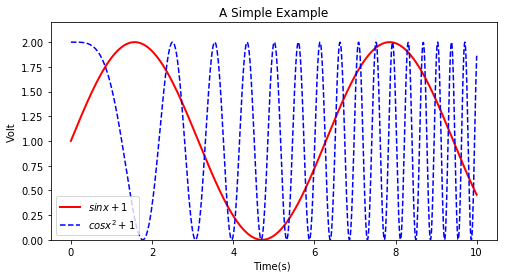np.random.seed(1000)
y=np.random.standard_normal(20)
print y
x=range(len(y))
print x
plt.plot(x,y)
plt.plot(y.cumsum(),'b',lw=1.5)
plt.plot(y.cumsum(),'ro',lw=1.5)
plt.show()
[-0.8044583   0.32093155 -0.02548288  0.64432383 -0.30079667  0.38947455
-0.1074373  -0.47998308  0.5950355  -0.46466753  0.66728131 -0.80611561
-1.19606983 -0.40596016 -0.18237734  0.10319289 -0.13842199  0.70569237
1.27179528 -0.98674733]
[0, 1, 2, 3, 4, 5, 6, 7, 8, 9, 10, 11, 12, 13, 14, 15, 16, 17, 18, 19]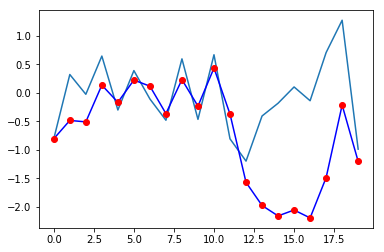np.random.seed(2000)
#y1=np.random.standard_normal((20,2))
y=np.random.standard_normal((20,2)).cumsum(axis=0)
print y
plt.figure(figsize=(9,4))
plt.subplot(121)
plt.plot(y[:,0],lw=1.5,label='1st')
plt.plot(y[:,0],'ro')
plt.grid(True)
plt.legend(loc=0)
plt.axis('tight')
plt.xlabel('index')
plt.ylabel('value')
plt.title('1st Data Set')
plt.subplot(122)
plt.bar(np.arange(len(y)),y[:,1],width=0.5,color='g',label='2nd')
plt.grid(True)
plt.legend(loc=0)
plt.axis('tight')
plt.xlabel('index')
plt.ylabel('value')
plt.title('2st Data Set')
[[ 1.73673761  1.89791391]
[-0.37003581  1.74900181]
[ 0.21302575 -0.51023122]
[ 0.35026529 -1.21144444]
[-0.27051479 -1.6910642 ]
[ 0.93922398 -2.76624806]
[ 1.74614319 -3.05703153]
[ 1.52519555 -3.22618757]
[ 2.62602999 -3.14367705]
[ 2.6216544  -4.8662353 ]
[ 3.67921082 -7.38414811]
[ 1.7685707  -6.07769276]
[ 2.19296834 -6.54686084]
[ 1.18689581 -7.46878388]
[ 1.81330034 -7.11160718]
[ 1.79458178 -6.89043591]
[ 2.49318589 -6.05592589]
[ 0.82754806 -8.95736573]
[ 0.77890953 -9.00274406]
[ 2.25424343 -9.51643749]]

Text(0.5,1,u'2st Data Set')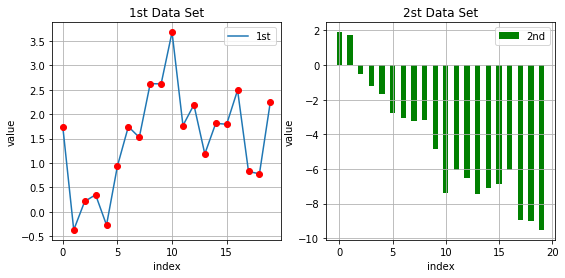pandas 的简单例子 (数据的读取、处理和探索) series(类似一维数组） dataFrame（相当于一张二维的表格，每一列都是一个Series）
import numpy as np
import pandas as pd
df=pd.DataFrame([10,20,30,40],columns=['numbers'],index=['a','b','c','d'])
df

numbersa10b20c30d40

df.index
Index([u'a', u'b', u'c', u'd'], dtype='object')

df.columns
Index([u'numbers'], dtype='object')

print df.ix['c']
print df.ix[['a','d']]
print df.ix[df.index[1:3]]
print df.sum()
print df.apply(lambda x:x**2)
numbers    30
Name: c, dtype: int64
numbers
a       10
d       40
numbers
b       20
c       30
numbers    100
dtype: int64
numbers
a      100
b      400
c      900
d     1600

df['floats']=(1.5,2.5,3.5,4.5)
df

numbersfloatsa101.5b202.5c303.5d404.5

df['names']=pd.DataFrame(['Yves','Guido','Feild','Fance'],index=['a','b','c','d'])
print df
df=df.append(pd.DataFrame({'numbers':100,'floats':5.75,'names':'Henry'},index=['Z']))
print df
   numbers  floats  names
a       10     1.5   Yves
b       20     2.5  Guido
c       30     3.5  Feild
d       40     4.5  Fance
floats  names  numbers
a    1.50   Yves       10
b    2.50  Guido       20
c    3.50  Feild       30
d    4.50  Fance       40
Z    5.75  Henry      100

df['floats']['a']
1.5

type(df)
pandas.core.frame.DataFrame

df['numbers']
a     10
b     20
c     30
d     40
Z    100
Name: numbers, dtype: int64

type(df['numbers'])
pandas.core.series.Series

import matplotlib.pyplot as plt
df['numbers'].cumsum().plot(style='r',lw=2.) #画numbers这列的累加图形
plt.xlabel('date')
plt.ylabel('value')
plt.show()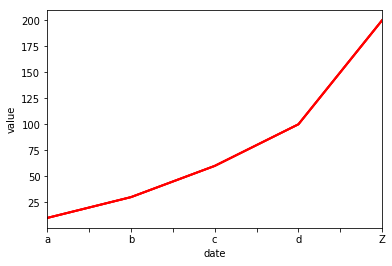import pandas as pd     #Series和DataFrame数据结构
s=pd.Series([1,2,3],index=['a','b','c'])
print s
d=pd.DataFrame([[1,2,3],[4,5,6]],columns=['a','b','c'])
print d
d2=pd.DataFrame(s)
print d2
print d.head()
a    1
b    2
c    3
dtype: int64
a  b  c
0  1  2  3
1  4  5  6
0
a  1
b  2
c  3
a  b  c
0  1  2  3
1  4  5  6

StatsModels 注重数据的统计建模分析，使pytohn有R语言的味道， StatsModels支持与pandas进行数据交互，强大的数据挖掘组合
from statsmodels.tsa.stattools import adfuller as ADF
from pandas.core import datetools
import numpy as np

(-11.253758305760554,
1.6868652157937374e-20,
0L,
99L,
{'1%': -3.4981980821890981,
'10%': -2.5825959973472097,
'5%': -2.8912082118604681},
31.149412019471782)

Scilit-Learn包括数据预处理、分类与预测、回归、聚类和模型分析
from sklearn import datasets #导入数据集
print(iris.data.shape)

from sklearn import svm #建立线性SVM分类器
clf=svm.LinearSVC()#建立线性SVM分类器
clf.fit(iris.data,iris.target)# 用数据训练模型
clf.predict([[5.0,3.6,1.5,0.25]])#训练好模型之后，输入新的数据进行预测
clf.coef_  #训练好模型的参数


(150L, 4L)

array([[ 0.18424073,  0.451224  , -0.80793865, -0.45071743],
[ 0.0533189 , -0.89005676,  0.40352144, -0.93828342],
[-0.85072726, -0.98671105,  1.38088737,  1.86538905]])

Keras 搭建神经网络 自编码器、循环神经网络、递归神经网络、卷积神经网络Gensim 处理语言方面的任务，如文本相似度计算、word2Vec等
数据探索
通过检验数据集的数据质量、绘制图表、计算某些特征量等手段，对样本数据集的结构和规律进行分析的过程。  有助于选择合适的数据预处理和建模方法。
数据质量分析
缺失值、异常值、不一致的值、重复数据及含有特殊符号的数据（#、￥、*）
缺失值的处理
缺失值产生的原因 1、信息暂时无法获取，或者信息获取代价太大 2、信息被遗漏 3、属性值不存在缺失值的影响 数据挖掘建模将丢失大量有用信息； 数据挖掘模型表现出的不确定性更加显著，规律难把握；包含空值的数据建模，导致不可靠的输出。缺失值分析 统计分析的方法，可以得到含有缺失值属性的个数。 删除记录、插补、不处理
异常值分析
简单统计量分析（最大值，最小值）、箱形图分析
import pandas as pd
catering_sale='data3/catering_sale.xls'  #餐饮数据
data.head()

销量日期2015-03-0151.02015-02-282618.22015-02-272608.42015-02-262651.92015-02-253442.1

data.describe()

销量count200.000000mean2755.214700std751.029772min22.00000025%2451.97500050%2655.85000075%3026.125000max9106.440000

import pandas as pd

catering_sale = 'data3/catering_sale.xls' #餐饮数据
data = pd.read_excel(catering_sale, index_col = u'日期') #读取数据，指定“日期”列为索引列

import matplotlib.pyplot as plt #导入图像库
plt.figure() #建立图像
p = data.boxplot(return_type='dict') #画箱线图，直接使用DataFrame的方法
x = p['fliers'].get_xdata() # 'flies'即为异常值的标签
y = p['fliers'].get_ydata()
y.sort() #从小到大排序，该方法直接改变原对象

#用annotate添加注释
#其中有些相近的点，注解会出现重叠，难以看清，需要一些技巧来控制。
for i in range(len(x)):
if i>0:
plt.annotate(y[i], xy = (x[i],y[i]), xytext=(x[i]+0.05 -0.8/(y[i]-y[i-1]),y[i]))
else:
plt.annotate(y[i], xy = (x[i],y[i]), xytext=(x[i]+0.08,y[i]))

plt.show() #展示箱线图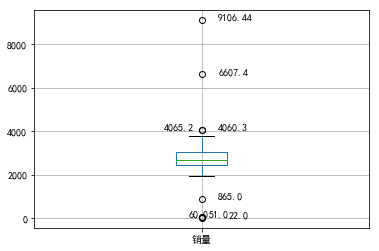数据特征分析
分布分析（定量数据的分布分析,定性数据的分布分析）、对比分析、统计量分析、周期性分析、贡献度分析、相关性分析，了解数据的规律和趋势，为数据挖掘的后续环节提供支持。
统计量分析：集中趋势度量（均值、中位数、众数）；离中趋势度量（极差、标准差、变异系数、四分位间距）；
# 统计量分析
import pandas as pd

catering_sale = 'data3/catering_sale.xls' #餐饮数据
data = pd.read_excel(catering_sale, index_col = u'日期') #读取数据，指定“日期”列为索引列
data = data[(data[u'销量'] > 400)&(data[u'销量'] < 5000)] #过滤异常数据
statistics = data.describe() #保存基本统计量

statistics.loc['range'] = statistics.loc['max']-statistics.loc['min'] #极差 最大值减最小值
statistics.loc['var'] = statistics.loc['std']/statistics.loc['mean'] #变异系数    比较两个或多个具有不同单位或波动幅度的数据集的离中趋势
statistics.loc['dis'] = statistics.loc['75%']-statistics.loc['25%'] #四分位数间距 值越大说明变异程度越大

print(statistics)
                销量
count   195.000000
mean   2744.595385
std     424.739407
min     865.000000
25%    2460.600000
50%    2655.900000
75%    3023.200000
max    4065.200000
range  3200.200000
var       0.154755
dis     562.600000

贡献度分析：A1-A7总盈利占85%
import pandas as pd

#初始化参数
dish_profit = 'data3/catering_dish_profit.xls' #餐饮菜品盈利数据
data = pd.read_excel(dish_profit, index_col = u'菜品名')
data = data[u'盈利'].copy()
data.sort_values(ascending = False)

import matplotlib.pyplot as plt #导入图像库
plt.figure()
data.plot(kind='bar')
plt.ylabel(u'盈利（元）')
p = 1.0*data.cumsum()/data.sum()
p.plot(color = 'r', secondary_y = True, style = '-o',linewidth = 2)
plt.annotate(format(p, '.4%'), xy = (6, p), xytext=(6*0.9, p*0.9), arrowprops=dict(arrowstyle="->", connectionstyle="arc3,rad=.2")) #添加注释，即85%处的标记。这里包括了指定箭头样式。
plt.ylabel(u'盈利（比例）')
plt.show()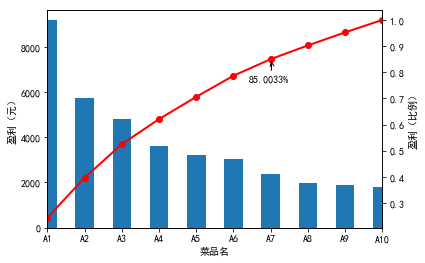相关性分析：相关系数

r=∑ni=1(xi−x¯)(yi−y¯)∑ni=1(xi−x¯)2∑ni=1(yi−y¯)2−−−−−−−−−−−−−−−−−−−−−−√

r

=

∑

i

=

1

n

(

x

i

−

x

¯

)

(

y

i

−

y

¯

)

∑

i

=

1

n

(

x

i

−

x

¯

)

2

∑

i

=

1

n

(

y

i

−

y

¯

)

2

r=\frac{\sum_{i=1}^n {(x_i-\bar x)(y_i-\bar y)}}{\sqrt{{\sum_{i=1}^n (x_i-\bar x)^2}{\sum_{i=1}^n(y_i-\bar y)^2}}}

import pandas as pd

catering_sale = 'data3/catering_sale_all.xls' #餐饮数据，含有其他属性
data = pd.read_excel(catering_sale, index_col = u'日期')

data.corr() #相关系数矩阵，即给出了任意两款菜式之间的相关系数
print data.corr()[u'百合酱蒸凤爪'] #只显示“百合酱蒸凤爪”与其他菜式的相关系数
print data[u'百合酱蒸凤爪'].corr(data[u'翡翠蒸香茜饺']) #计算“百合酱蒸凤爪”与“翡翠蒸香茜饺”的相关系数
            百合酱蒸凤爪  翡翠蒸香茜饺  金银蒜汁蒸排骨  乐膳真味鸡  蜜汁焗餐包  生炒菜心  铁板酸菜豆腐  香煎韭菜饺  香煎罗卜糕  \
日期
2015-01-01      17       6        8     24   13.0    13      18     10     10
2015-01-02      11      15       14     13    9.0    10      19     13     14
2015-01-03      10       8       12     13    8.0     3       7     11     10
2015-01-04       9       6        6      3   10.0     9       9     13     14
2015-01-05       4      10       13      8   12.0    10      17     11     13

原汁原味菜心
日期
2015-01-01      27
2015-01-02      13
2015-01-03       9
2015-01-04      13
2015-01-05      14
百合酱蒸凤爪     1.000000
翡翠蒸香茜饺     0.009206
金银蒜汁蒸排骨    0.016799
乐膳真味鸡      0.455638
蜜汁焗餐包      0.098085
生炒菜心       0.308496
铁板酸菜豆腐     0.204898
香煎韭菜饺      0.127448
香煎罗卜糕     -0.090276
原汁原味菜心     0.428316
Name: 百合酱蒸凤爪, dtype: float64
0.00920580305184

数据预处理
数据预处理占到60%， 数据清洗、数据集成（属性冗余问题），数据变换、数据规约。
数据清洗主要包括：缺失值处理（均值、中位数、众数插补，最近临插补、回归方法、插值法）、异常值处理（删除、视为缺失值、平均值修正）。

y=a0+a1x+a2x2+...+an−1xn−1

y

=

a

0

+

a

1

x

+

a

2

x

2

+

.

.

.

+

a

n

−

1

x

n

−

1

y= a_0+a_1x+a_2x^2+...+a_{n-1}x^{n-1} lagrange插值法：
#拉格朗日插值代码
#拉格朗日插值代码
import pandas as pd #导入数据分析库Pandas
from scipy.interpolate import lagrange #导入拉格朗日插值函数

inputfile = 'data4/catering_sale.xls' #销量数据路径
outputfile = './sales.xls' #输出数据路径

#data[u'销量'][(data[u'销量'] < 400) | (data[u'销量'] > 5000)] = None #过滤异常值，将其变为空值
row_indexs = (data[u'销量'] < 400) | (data[u'销量'] > 5000)  #得到过滤数据的索引
data.loc[row_indexs,u'销量'] = None  #过滤数据

#自定义列向量插值函数
#s为列向量，n为被插值的位置，k为取前后的数据个数，默认为5
def ployinterp_column(s, n, k=5):
y = s[list(range(n-k, n)) + list(range(n+1, n+1+k))] #取数
y = y[y.notnull()] #剔除空值
return lagrange(y.index, list(y))(n) #插值并返回拉格朗日插值结果

#逐个元素判断是否需要插值
for i in data.columns:
for j in range(len(data)):
if (data[i].isnull())[j]: #如果为空即插值。
#       data[i][j] = ployinterp_column(data[i], j)
data.loc[j,i] = ployinterp_column(data[i], j)
data.to_excel(outputfile) #输出结果，写入文件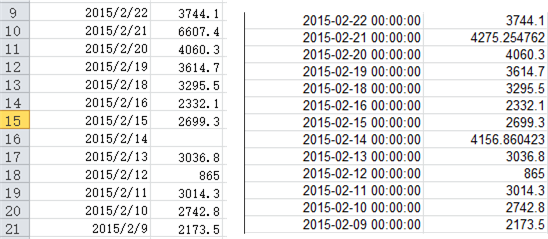数据归一化：
#数据规范化
import pandas as pd
import numpy as np

datafile = 'data4/normalization_data.xls' #参数初始化

(data - data.min())/(data.max() - data.min()) #最小-最大规范化
(data - data.mean())/data.std() #零-均值规范化
data/10**np.ceil(np.log10(data.abs().max())) #小数定标规范化

012300.0780.5210.6020.286310.144-0.600-0.5210.224520.095-0.4570.468-0.128330.0690.5960.6950.105440.1900.5270.6910.205150.1010.4030.4700.248760.1460.4130.4350.2571

连续属性离散化：  等宽离散化: 将属性的值域分成相同宽度的区间
等频离散化：间相同数量的记录放进每个区间
基于聚类
#-*- coding: utf-8 -*-

import pandas as pd

datafile = 'data4/discretization_data.xls' #参数初始化
data = data[u'肝气郁结证型系数'].copy()

k = 4
#等宽离散化
d1 = pd.cut(data, k, labels = range(k)) #等宽离散化，各个类比依次命名为0,1,2,3

#等频率离散化
w = [1.0*i/k for i in range(k+1)]  #[0.0, 0.25, 0.5, 0.75, 1.0]
w = data.describe(percentiles = w)[4:4+k+1] #使用describe函数自动计算分位数
w = w*(1-1e-10)
d2 = pd.cut(data, w, labels = range(k))

from sklearn.cluster import KMeans #引入KMeans
kmodel = KMeans(n_clusters = k, n_jobs = 4) #建立模型，n_jobs是并行数，一般等于CPU数较好
kmodel.fit(data.values.reshape((len(data), 1))) #训练模型
c = pd.DataFrame(kmodel.cluster_centers_).sort_values(0) #输出聚类中心，并且排序（默认是随机序的）
w = c.rolling(window=2,center=False).mean().iloc[1:] #相邻两项求中点，作为边界点
#w = pd.DataFrame.rolling(window=2,center=False).mean()
w =  + list(w) + [data.max()] #把首末边界点加上
d3 = pd.cut(data, w, labels = range(k))

def cluster_plot(d, k): #自定义作图函数来显示聚类结果
import matplotlib.pyplot as plt

plt.figure(figsize = (8, 3))
for j in range(0, k):
plt.plot(data[d==j], [j for i in d[d==j]], 'o')

plt.ylim(-0.5, k-0.5)
return plt

cluster_plot(d1, k).show()

cluster_plot(d2, k).show()
cluster_plot(d3, k).show()
0    0.056
1    0.488
2    0.107
3    0.322
4    0.242
5    0.389
6    0.246
7    0.330
8    0.257
9    0.205
Name: 肝气郁结证型系数, dtype: float64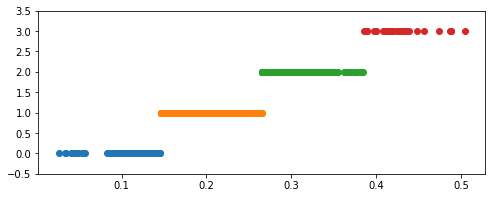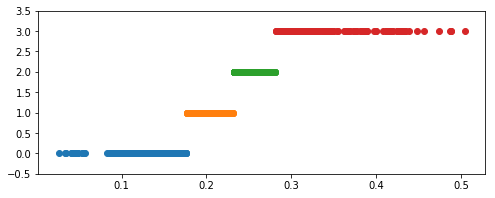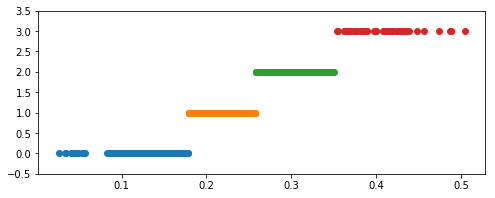#主成分分析 降维
import pandas as pd

#参数初始化
inputfile = 'data4/principal_component.xls'
outputfile = './dimention_reducted.xls' #降维后的数据

from sklearn.decomposition import PCA

pca = PCA()
pca.fit(data)
print pca.components_ #返回模型的各个特征向量
print pca.explained_variance_ratio_ #返回各个成分各自的方差百分比
[[ 0.56788461  0.2280431   0.23281436  0.22427336  0.3358618   0.43679539
0.03861081  0.46466998]
[ 0.64801531  0.24732373 -0.17085432 -0.2089819  -0.36050922 -0.55908747
0.00186891  0.05910423]
[-0.45139763  0.23802089 -0.17685792 -0.11843804 -0.05173347 -0.20091919
-0.00124421  0.80699041]
[-0.19404741  0.9021939  -0.00730164 -0.01424541  0.03106289  0.12563004
0.11152105 -0.3448924 ]
[-0.06133747 -0.03383817  0.12652433  0.64325682 -0.3896425  -0.10681901
0.63233277  0.04720838]
[ 0.02579655 -0.06678747  0.12816343 -0.57023937 -0.52642373  0.52280144
0.31167833  0.0754221 ]
[-0.03800378  0.09520111  0.15593386  0.34300352 -0.56640021  0.18985251
-0.69902952  0.04505823]
[-0.10147399  0.03937889  0.91023327 -0.18760016  0.06193777 -0.34598258
-0.02090066  0.02137393]]
[  7.74011263e-01   1.56949443e-01   4.27594216e-02   2.40659228e-02
1.50278048e-03   4.10990447e-04   2.07718405e-04   9.24594471e-05]

pca=PCA(3)
pca.fit(data)
low_d=pca.transform(data)
low_d
array([[  8.19133694,  16.90402785,   3.90991029],
[  0.28527403,  -6.48074989,  -4.62870368],
[-23.70739074,  -2.85245701,  -0.4965231 ],
[-14.43202637,   2.29917325,  -1.50272151],
[  5.4304568 ,  10.00704077,   9.52086923],
[ 24.15955898,  -9.36428589,   0.72657857],
[ -3.66134607,  -7.60198615,  -2.36439873],
[ 13.96761214,  13.89123979,  -6.44917778],
[ 40.88093588, -13.25685287,   4.16539368],
[ -1.74887665,  -4.23112299,  -0.58980995],
[-21.94321959,  -2.36645883,   1.33203832],
[-36.70868069,  -6.00536554,   3.97183515],
[  3.28750663,   4.86380886,   1.00424688],
[  5.99885871,   4.19398863,  -8.59953736]])

挖掘建模
分类与预测（回归分析、决策树（ID3）、神经网络、贝叶斯网络、支持向量机）  聚类分析  关联规则（Apriori）  时序模式  偏差检测
ID3算法核心是在决策树的各级节点上，使用信息增益方法作为属性的选择标准，来帮助确定生成每个节点时所采用的合适属性。

I(s1,s2,...,sm)=−∑i=1mPilog2(Pi)

I

(

s

1

,

s

2

,

.

.

.

,

s

m

)

=

−

∑

i

=

1

m

P

i

l

o

g

2

(

P

i

)

I(s_1,s_2,...,s_m)=-\sum_{i=1}^m P_ilog_2(P_i)

m表示属性值的个数，

si

s

i

$s_i$ 是某个属性值的样本个数,

Pi

P

i

$P_i$ 可以用

siS

s

i

S

$\frac{s_i}S$,S是s样本数据的集合。
总信息熵：销售数量高的数据18，低的数据16,

I(18,16)=−1834log21834−1634log21634

I

(

18

,

16

)

=

−

18

34

l

o

g

2

18

34

−

16

34

l

o

g

2

16

34

$I(18,16)=- \frac{18}{34} log_2 \frac{18}{34}- \frac{16}{34} log_2 \frac{16}{34}$
是否周末属性：是的条件下，销售高的记录11，低为3，表示（11，3）；不是的条件下，销售高记录7，低为13，表示（7，13）；
是否周末的属性的信息熵增益：

I(18,16)−(1434I(11,3)+2034I(7,13))

I

(

18

,

16

)

−

(

14

34

I

(

11

,

3

)

+

20

34

I

(

7

,

13

)

)

$I(18,16)-(\frac{14}{34}I(11,3)+\frac{20}{34}I(7,13))$

#使用ID3决策树算法预测销量高低
import pandas as pd

#参数初始化
inputfile = 'data5/sales_data.xls'
data = pd.read_excel(inputfile, index_col = u'序号') #导入数据

#数据是类别标签，要将它转换为数据
#用1来表示“好”、“是”、“高”这三个属性，用-1来表示“坏”、“否”、“低”
data[data == u'好'] = 1
data[data == u'是'] = 1
data[data == u'高'] = 1
data[data != 1] = -1
x = data.iloc[:,:3].as_matrix().astype(int) #前三个属性的矩阵
y = data.iloc[:,3].as_matrix().astype(int) #销量的矩阵

from sklearn.tree import DecisionTreeClassifier as DTC
dtc = DTC(criterion='entropy') #建立决策树模型，基于信息熵
dtc.fit(x, y) #训练模型

#导入相关函数，可视化决策树。
#导出的结果是一个dot文件，需要安装Graphviz才能将它转换为pdf或png等格式。
from sklearn.tree import export_graphviz
x = pd.DataFrame(x)
from sklearn.externals.six import StringIO
x = pd.DataFrame(x)
with open("tree.dot", 'w') as f:
f = export_graphviz(dtc, feature_names = x.columns, out_file = f)

   天气 是否周末 是否有促销 销量
序号
1   坏    是     是  高
2   坏    是     是  高
3   坏    是     是  高
4   坏    否     是  高
5   坏    是     是  高
天气 是否周末 是否有促销 销量
序号
1   -1    1     1  1
2   -1    1     1  1
3   -1    1     1  1
4   -1   -1     1  1
5   -1    1     1  1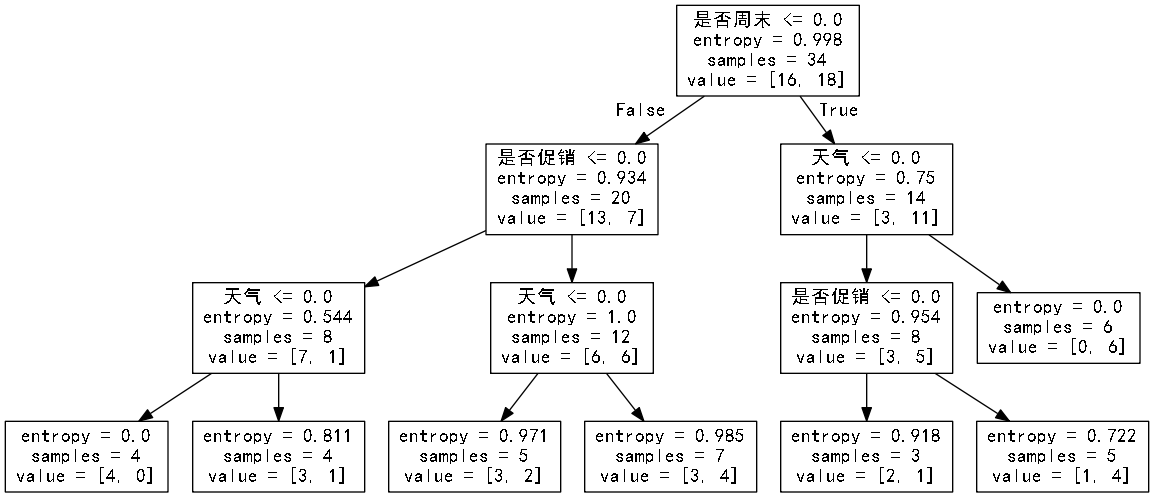value=[0,6]代表0个低，6个高
Apriori算法： 找最大K项频繁集 没一项的支持度 p（a）=7/10, 支持度为0.2（支持度计数为2）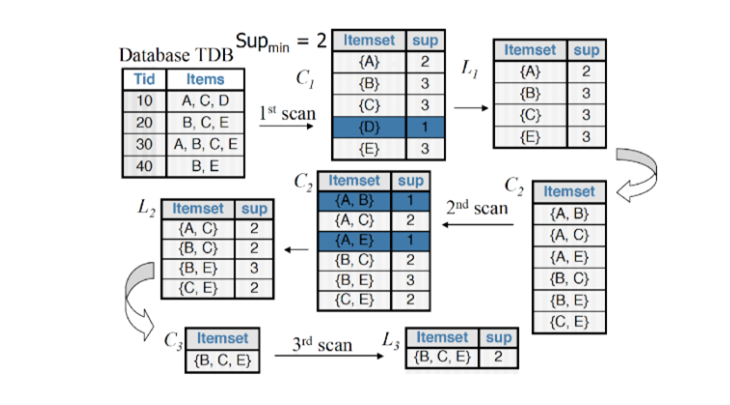#使用Apriori算法挖掘菜品订单关联规则
import pandas as pd
from apriori import * #apriori函数

outputfile = './apriori_rules.xls' #结果文件

print(u'\n转换原始数据至0-1矩阵...')
ct = lambda x : pd.Series(1, index = x[pd.notnull(x)]) #转换0-1矩阵的过渡函数
b = map(ct, data.as_matrix()) #用map方式执行
data = pd.DataFrame(list(b)).fillna(0) #实现矩阵转换，空值用0填充
print(u'\n转换完毕。')
del b #删除中间变量b，节省内存

support = 0.2 #最小支持度 同时发生的概率
confidence = 0.5 #最小置信度  A发生则B发生的概率
ms = '---' #连接符，默认'--'，用来区分不同元素，如A--B。需要保证原始表格中不含有该字符

find_rule(data, support, confidence, ms).to_excel(outputfile) #保存结果
   0  1    2    3
0  a  c    e  NaN
1  b  d  NaN  NaN
2  b  c  NaN  NaN
3  a  b    c    d
4  a  b  NaN  NaN
5  b  c  NaN  NaN
6  a  b  NaN  NaN
7  a  b    c    e
8  a  b    c  NaN
9  a  c    e  NaN

转换原始数据至0-1矩阵...

转换完毕。

正在进行第1次搜索...
数目：6...

正在进行第2次搜索...
数目：3...

正在进行第3次搜索...
数目：0...

结果为：
support  confidence
e---a          0.3    1.000000
e---c          0.3    1.000000
c---e---a      0.3    1.000000
a---e---c      0.3    1.000000
a---b          0.5    0.714286
c---a          0.5    0.714286
a---c          0.5    0.714286
c---b          0.5    0.714286
b---a          0.5    0.625000
b---c          0.5    0.625000
b---c---a      0.3    0.600000
a---c---b      0.3    0.600000
a---b---c      0.3    0.600000
a---c---e      0.3    0.600000

展开全文• 词法分析器的任务是读入源程序，对其进行一定的切分，得到记号流 对于字符流和记号流之间的区别，下面给出一个例子来说明 if (x &gt; 5) y = "hello"; else z = 1; 对面上面这段程序，在词法分析器...
在编译原理介绍中，我们已经对前端的工作有了大致的了解词法分析器的任务是读入源程序，对其进行一定的切分，得到记号流
对于字符流和记号流之间的区别，下面给出一个例子来说明
if (x > 5)
y = "hello";
else
z = 1;

对面上面这段程序，在词法分析器的眼中，是这样表示的： i, f, " ", (, x, " ",>, " ", 5, ), \n, " “,” ", y, " ", =, …
可见词法分析器看到的字符流和我们眼中的字符流是不一样的。 而词法分析器的任务就是对字符进行 切分。将 i 和 f 并在一起做为一个 if 关键字，去除掉没有意义的空格等等对于像IF，LPAREN，IDENT等的单词，我们都称为记号
在记号中，有一些记号是没有属性的，类似于 IF 之类的关键字， IDENT(x) 之类的标识符需要表明其中的元素具体是什么
记号的数据结构定义如下：
enum kind {IF, LPAREN, ID, INTLIT, ...};
struct token{
enum kind k;
char *lexname;
}

因此，对于语句 if (x > 5) 可以转变为
token {
k = IF;
lexname = 0; //0 表示没有赋任何的值
}
token {
k = LPAREN;
laxname = 0;
}
token {
k = ID;
laxname = "x";
}
....

通过上面的学习，我们可以知道词法分析器的任务是将字符流转化为记号流
字符流：和被编译的语言密切相关（ASCII（C）， Unicode（Java, Swift）， or …）记号流：编译器内部定义的数据结构，编码所识别出的词法单元
展开全文编译原理
• 随机森林：随机森林是一种多功能的机器学习算法，能够执行回归和分类的任务。同时，它也是一种数据降维手段，用于处理缺失值、异常值以及其他数据探索中的重要步骤，并取得了不错的成效。 二、加载数据 ...
一、导入模块
%matplotlib inline             #将生成的图片嵌入网页中
import matplotlib.pyplot as plt
from sklearn import datasets
from sklearn.feature_selection import SelectKBest,f_regression
from sklearn.linear_model import LinearRegression              #导入先行回归模型
from sklearn.svm import SVR                                    #导入svm模型
from sklearn.ensemble import RandomForestRegressor             #导入随机森林模型
我们用到的模块有matplotlib可视化，还有sklearn机器学习模块，其中也用到了LinearRegression线性回归模型、SVM（支持向量机）回归模型和RandomForestRegressor随机森林模型。
线性回归：，线性回归(Linear Regression)是利用称为线性回归方程的最小平方函数对一个或多个自变量和因变量之间关系进行建模的一种回归分析。这种函数是一个或多个称为回归系数的模型参数的线性组合。只有一个自变量的情况称为简单回归,大于一个自变量情况的叫做多元回归。支持向量机：支持向量机（SVM，还支持矢量网络）是与相关的学习算法有关的监督学习模型，可以分析数据，识别模式，用于分类和回归分析。给定一组训练样本，每个标记为属于两类，一个SVM训练算法建立了一个模型，分配新的实例为一类或其他类，使其成为非概率二元线性分类。随机森林：随机森林是一种多功能的机器学习算法，能够执行回归和分类的任务。同时，它也是一种数据降维手段，用于处理缺失值、异常值以及其他数据探索中的重要步骤，并取得了不错的成效。
二、加载数据
boston_data = datasets.load_boston()
print(x_full.shape)
print(y.shape)

#输出：
(506, 13)
(506,)
三、转换和分析
selector = SelectKBest(f_regression,k=1)            #选出相关性最强的SelectKBest类作为特征
selector.fit(x,y)                                   #采用fit()方法进行数据拟合
x = x_full[:,selector.get_support()]                     #采用get_support()将数据缩减成一个向量，即数据降维
print(x.shape)

#输出：
(506, 1)
在这一步，我们用SelectKBest类选出相关性最强的一个特征，也就是一个向量。采用fit()方法将数据拟合，然后用get_support()将数据缩减成一个向量，即数据降维。
四、可视化
def plot_scatter(x,y,R=None):
plt.scatter(x,y,s=32,marker='o',facecolors='blue')
if R is not None:
plt.scatter(x,R,color='red',linewidth=0.5)
plt.show()

plot_scatter(x,y)
由于目标是一个向量，所以我们可以看看输入（特征）和输出（房价）之间是都存在线性关系。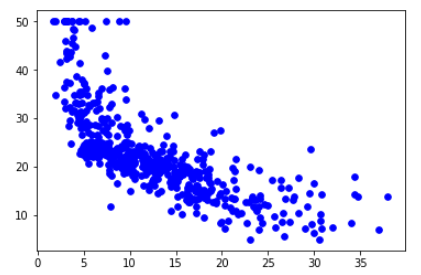根据输出的结果，我们可以看到随着x的增加，y在减少，但是这种变化比率不是恒定的。这是一个非线性情况，我们可以利用一个回归模型进一步将它可视化。
五、模型分析
#线性回归模型
regressor = LinearRegression(normalize=True).fit(x,y)
plot_scatter(x,y,regressor.predict(x))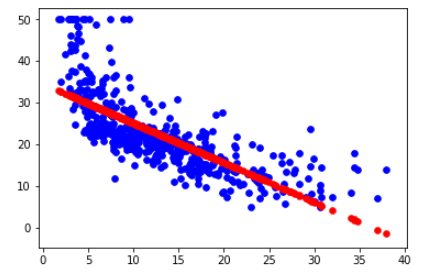这一步，我们创建并训练了一个线性回归模型，红色画出了输入和输出之间的最佳线性关系。很显然我们看到这个效果并不是很好，它只是一个近似值。
接下来我们看看支持向量机和随机森林的模型效果。
#SVM回归模型
regressor = SVR().fit(x,y)
plot_scatter(x,y,regressor.predict(x))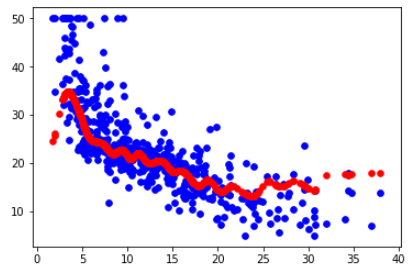支持向量机是一种用来解决非线性问题的模型，可以看到在这种情况下，支持向量机的效果比线性回归的效果更好一点。
最后再看看随机森林。
#随机森林回归模型
regressor =RandomForestRegressor().fit(x,y)
plot_scatter(x,y,regressor.predict(x))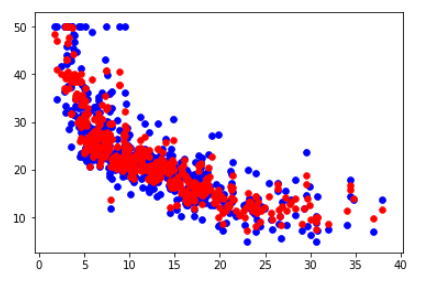我们看到这种情况下利用随机森林的效果比前面两个好多了，随机森林是一种四栋解决非线性问题的模型。
展开全文python 随机森林 svm
• 查看了很多相关crontab定时任务的博客，觉得很有道理，但也很乱！踩了不少坑之后，下定决心写一篇平滑度过Crontab入门的博客，不当之处万望指教。 问题： 我有一个叫test4.py的Python脚本，需要定时执行，实时更新...
• MapReduce 运行的时候，会通过 Mapper 运行的任务读取 HDFS 中的数据文件，然后调用自己的方法，处理数据，最后输出。Reducer 任务会接收 Mapper 任务输出的数据，作为自己的输入数据，调用自己的方法，最后输出到 ...
• FreeRTOS任务相关的代码大约占总代码的一半左右，这些代码都在为一件事情而努力，即找到优先级最高的就绪任务，并使之获得CPU运行权。任务切换是这一过程的直接实施者，为了更快的找到优先级最高的就绪任务任务...
• “2-1-1”丢包分析 打开 Cygwin.terminal ，输入 startxwin 进入 Cygwin 的 xwindow 界面，输入 source bashrc 进行环境变量的配置；输入 cd test 切换目录到 test 文件夹，输入 ns example2.tcl 进行仿真。 ...ns2 丢包 cygwin
• 本文主要介绍了文本情感分析的主要任务，包括词级别的情感分析、句子级情感分析以及属性级情感分析任务的介绍，同时介绍了华为云在这三个任务上的一些进展。用户可以直接在EI体验空间小程序体验这些功能。 1基本...人工智能 机器学习 自然语言 华为云 程序员
• 首先使用maven搭建一个Springboot 项目。 添加依赖到pom.xml中 &lt;dependencies&gt; &lt;dependency&gt; &lt;groupId&gt;org.springframework.boot&lt;/groupId&......springboot内核 鸿蒙 鸿蒙源码 鸿蒙系统
• 需求分析 为了开发出真正满足用户需求的软件产品,首先必须... 需求分析是软件定义时期的最后一个阶段,它的基本任务是准确地回答“系统必须做什么?”这个问题 。 虽然在可行性研究阶段已经粗略地了解了用户的需求,...软件工程
• 情感分析是NLP中的一大分支，无论在学术界还是工业界都有广泛的研究，在SemEval语义分析会议中有单独的情感分析任务分支，其中最难的一个任务叫做ABSA：Aspect-Based Sentiment Analysis，面向方面的情感分析。...
• 最近公司有要搭建一个分布式定时任务平台的需求，并把这个任务交给了我。  首先是作了一番分析了解，...分布式
• 以上是比较通用的分析过程，本来还涉及到结合c代码的分析，跟具体的例子结合太紧密，就不赘述了，有一点可以提一下，想追溯函数调用过程中某一参数 的运行值，可能会在调用者的栈中，也可能会在调用者的调用者的栈中...汇编 c shell 优化
• Linux 是一个多用户、多任务的操作系统，我们应该了解单用户多任务和多用户多任务的概念。单用户多任务：比如我们以beinan 登录系统，进入系统后，我要打开gedit 来写文档，但在写文档的过程中，我感觉少点音乐，...linux 用户 用户组
• 用卷积神经网络对句子分类  ...针对句子级别的分类任务，我们使用卷积神经网络（CNN）结合预训练的词向量做了一系列的实验。我们证明一个少量调节超参数的简单CNN模型结合静态词向量可以在很多基准上取android AsyncTask Handlerandroid asynctask api大数据 hadoop集群 mapreduce 性能优化 hadoop
• 作者丨邴立东、李昕、李正、彭海韵、许璐单位丨阿里巴巴达摩院等任务简介我们略过关于 sentiment analysis 重要性的铺陈，直接进入本文涉及的任务。先上例子，对于一句餐馆评论：...
• 接下继续执行的就是Worker上的Executor进程了，本文继续分析整个Executor的启动与任务提交流程 Spark-submit提交一个任务到集群通过的是Spark-submit 通过启动脚本的方式启动它的主类，这里以WordCount为例子 `...spark 集群 云计算 大数据
• 目标情感分析任务（Targeted Sentiment Analysis, TSA）旨在提取目标实体并对其进行情感分类。因此可以理解为命名实体识别（NER）和情感分析（SA）两个任务的联合学习。例如，给出一句“ESPN调查显示迈克尔乔丹是最...
• 宽带离网用户分析任务介绍　接下来把几个研究生阶段的项目中所用到的关键技术分别做介绍，一来对这些项目的技术做个整理，理清思路，二来也为接下来的应聘做准备。这里先介绍研一做的第一个项目——宽带离网用户分析...数据挖掘
•源代码 Mapper SORT
• 在虚拟机栈分析时，就可以知道线程任务是由哪个线程工厂产生的。 第7个参数: handler 表示执行拒绝策略的对象。当超过第5个参数workQueue的任务缓存区上限且线程达到了maximumPoolSize的时候，就可以通过该策略处理...
• 产品需求分析与市场分析方法汇总（SWOT+PDCA+波士顿矩阵BCG+5W2H分析法+STAR关键事件分析法+目标管理SMART+时间管理紧急重要矩阵+WBS任务分解法） 产品需求分析与市场分析方法汇总 ... 一、KANO模型 ......